A Brief Introduction to Modern Time Series

Definition A time series is a random function xt of an argument t in a set T. In other words, a time series is a family of random variables ..., xt-1, xt, xt+1, ... corresponding to all elements in the set T, where T is supposed to be a denumerable, infinite set.

Definition An observed time series {xt | t e To Ė T} is regarded as a part of one realization of a random function xt. An infinite set of possible realizations which might have been observed is called an ensemble.

To put things more rigorously, the time series (or random function) is a real function x(w,t) of the two variables w and t, where wÎW and tÎT. If we fix the value of w, we have a real function x(t|w) of the time t, which is a realization of the time series. If we fix the value of t, then we have a random variable x(w|t). For a given point in time there is a probability distribution over x. Thus a random function x(w,t) can be regarded as either a family of random variables or as a family of realizations.

Definition We define the distribution function of the random variable w given t0 as P{x(w|to) Ģ x} =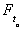(x). Similarly we can define the joint distribution for n random variables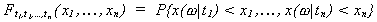The points which distinguish time series analysis from ordinary statistical analyses are the following
(1) The dependency among observations at different chronological points in time plays an essential role. In other words, the order of observations is important. In ordinary statistical analysis it is assumed that the observations are mutually independent.
(2) The domain of t is infinite.
(3) We have to make an inference from one realization. The realization of the random variable can be observed only once at each point in time. In multivariate analysis we have many observations on a finite number of variables. This critical difference necessitates the assumption of stationarity.

Definition The random function xt is said to be strictly stationary if all the finite dimensional distribution functions defining xt remain the same even if the whole group of points t1, t2, ..., tn is shifted along the time axis. That is, if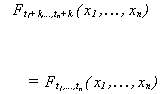for any integers t1, t2, ..., tn and k. Graphically, one could picture the realization of a strictly stationary series as having not only the same level in two different intervals, but also the same distribution function, right down to the parameters which define it.
The assumption of stationarity makes our lives simpler and less costly. Without stationarity we would have to sample the process frequently at each time point in order to build up a characterization of the distribution functions in the earlier definition. Stationarity means that we can confine our attention to a few of the simplest numerical functions, i.e., the moments of the distributions. The central moments are given by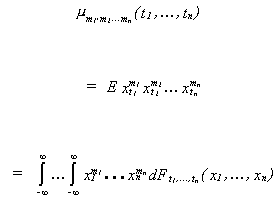Definition (i) The mean value of the time series {xt} is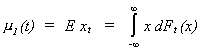i.e., the first order moment.
(ii) The autocovariance function of {xt} is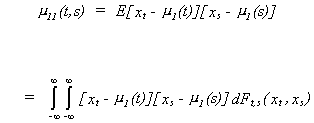i.e., the second moment about the mean. If t=s then you have the variance of xt. We will use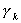to denote the autocovariance of a stationary series, where k denotes the difference between t and s.
(iii) The autocorrelation function (ACF) of {xt} is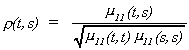We will use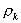to denote the autocorrelation of a stationary series, where k denotes the difference between t and s.
(iv) The partial autocorrelation (PACF), fkk, is the correlation between zt and zt+k after removing their mutual linear dependence on the intervening variables zt+1, zt+2, ..., z t+k-1.
One simple way to compute the partial autocorrelation between zt and zt+k is to run the two regressions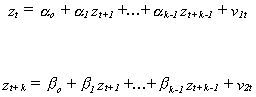then compute the correlation between the two residual vectors. Or, after measuring the variables as deviations from their means, the partial autocorrelation can be found as the LS regression coefficient on zt in the model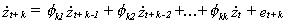10

where the dot over the variable indicates that it is measured as a deviation from its mean.
(v) The Yule-Walker equations provide an important relationship between the partial autocorrelations and the autocorrelations. Multiply both sides of equation 10 by z t+k-j and take expectations. This operation gives us the following difference equation in the autocovariances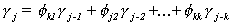or, in terms of the autocorrelations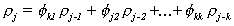This seemingly simple representation is really a powerful result. Namely, for j=1,2, ..., k we can write the full system of equations, known as the Yule-Walker equations,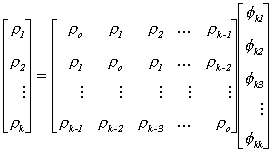From linear algebra you know that the matrix of r's is of full rank. Therefore it is possible to apply Cramer's rule successively for k=1,2,... to solve the system for the partial autocorrelations. The first three are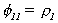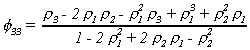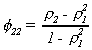We have three important results on strictly stationary series.

First, if {xt} is strictly stationary and E{xt}2 < Ĩ then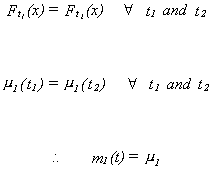The implication is that we can use any finite realization of the sequence to estimate the mean.
Second, if {xt} is strictly stationary and E{xt}2 < Ĩ then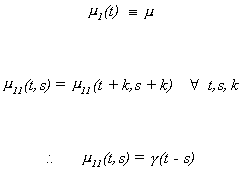The implication is that the autocovariance depends only on the difference between t and s, not their chronological point in time. We could use any pair of intervals in the computation of the autocovariance as long as the time between them was constant. And we can use any finite realization of the data to estimate the autocovariances.
Thirdly, the autocorrelation function in the case of strict stationarity is given by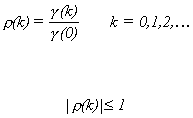The implication is that the autocorrelation depends only on the difference between t and s as well, and again they can be estimated by any finite realization of the data.

If our goal is to estimate parameters which are descriptive of the possible realizations of the time series, then perhaps strict stationarity is too restrictive. For example, if the mean and covariances of xt are constant and independent of the chronological point in time, then perhaps it is not important to us that the distribution function be the same for different time intervals.

Definition A random function is stationary in the wide sense (or weakly stationary, or stationary in Khinchin's sense, or covariance stationary) if m 1(t) šm and m11(t,s) =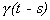.

Strict stationarity does not in itself imply weak stationarity. Weak stationarity does not imply strict stationarity. Strict stationarity with E{xt}2 < Ĩ
implies weak stationarity.

Ergodic theorems are concerned with the question of the necessary and sufficient conditions for making inference from a single realization of a time series. Basically it boils down to assuming weak stationarity.

Theorem If {xt} is weakly stationary with mean m and covariance function, then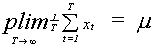That is, for any given e > 0 and h > 0 there exists some number To such that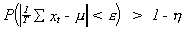for all T > To, if and only if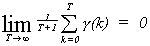This necessary and sufficient condition is that the autocovariances die out, in which case the sample mean is a consistent estimator for the population mean.

Corollary If {xt} is weakly stationary with E{xt+kxt}2 < Ĩ for any t, and E{xt+kxtxt+s+kxt+s} is independent of t for any integer s, then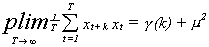if and only if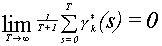where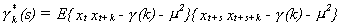A consequence of the corollary is the assumption that xtxt+k is weakly stationary. The Ergodic Theorem is no more than a law of large numbers when the observations are correlated.

One might ask at this point about the practical implications of stationarity. The most common application of use of time series techniques is in modelling macroeconomic data, both theoretic and atheoretic. As an example of the former, one might have a multiplier- accelerator model. For the model to be stationary, the parameters must have certain values. A test of the model is then to collect the relevant data and estimate the parameters. If the estimates are not consistent with stationarity, then one must rethink either the theoretical model or the statisticla model, or both.

We now have enough machinery to begin to talk about the modeling of univariate time series data. There are four steps in the process.
1. building models from theoretical and/or experiential knowledge
2. identifying models based on the data (observed series)
3. fitting the models (estimating the parameters of the model(s))
4. checking the model
If in the fourth step we are not satisfied we return to step one. The process is iterative until further checking and respecification yields no further improvement in results. DiagrammaticallyDefinition Some simple operations include the following:
The backshift operator Bxt = xt-1
The forward operator Fxt = xt+1
The difference operator Ņ = 1 - B
Ņxt = xt - xt-1
The difference operator behaves in a fashion consistent with the constant in an infinite series. That is, its inverse is the limit of an infinite sum. Namely, Ņ-1 = (1-B)-1 = 1/(1-B) = 1+B+B2+ ...
The integrate operator S = Ņ -1
Since it is the inverse of the difference operator, the integrate operator serves to construct the sum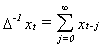.

MODEL BUILDING
In this section we offer a brief review of the most common sort of time series models. On the basis of one's knowledge of the data generating process one picks a class of models for identification and estimation from the possibilities which follow.

Autoregressive Models

Definition Suppose that Ext = m is independent of t. A model such as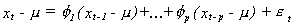with the characteristics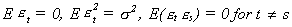is called the autoregressive model of order p, AR(p).

Definition If a time dependent variable (stochastic process) {xt} satisfies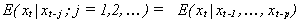then {xt} is said to satisfy the Markov property. On the LHS the expectation is conditioned on the infinite history of xt. On the RHS it is conditioned on only part of the history. From the definitions, an AR(p) model is seen to satisfy the Markov property.
Using the backshift operator we can write our AR model as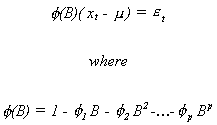Theorem A necessary and sufficient condition for the AR(p) model to be stationary is that all of the roots of the polynomial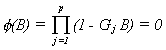lie outside the unit circle.

Example 1
Consider the AR(1)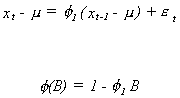The only root of 1 - f1B = 0 is B = 1/f1. The condition for stationarity requires that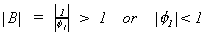.

If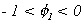then the observed series will appear very frenetic. E.g., consider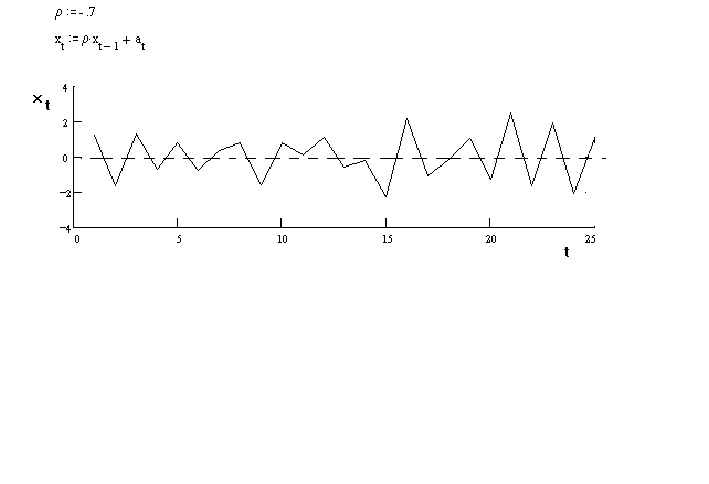in which the white noise term has a normal distribution with a zero mean and a variance of one. The observations switch sign with almost every observation.

If, on the other hand,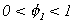then the observed series will be much smoother.

E.g.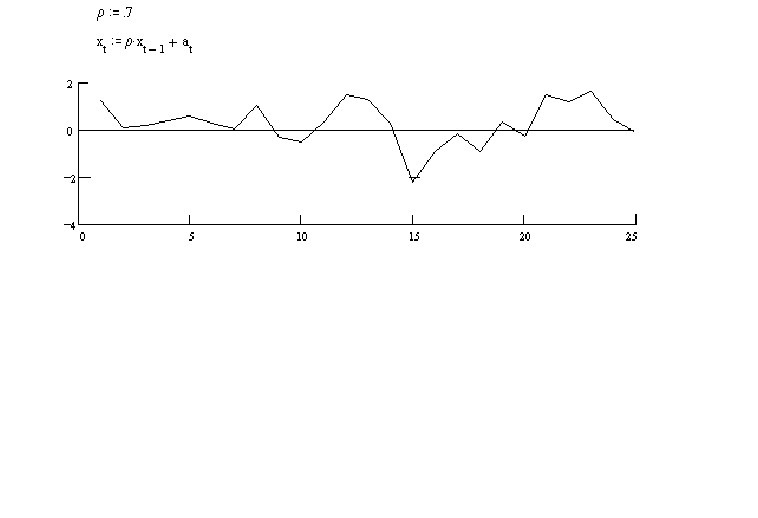In this series an observation tends to be above 0 if its predecessor was above zero.
The variance of et is se2 for all t. The variance of xt, when it has zero mean, is given by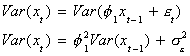Since the series is stationary we can write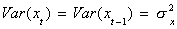. Hence,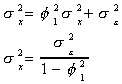The autocovariance function of an AR(1) series is, supposing without loss of generality m=0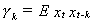To see what this looks like in terms of the AR parameters we will make use of the fact that we can write xt as follows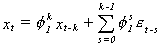Multiplying by xt-k and taking expectations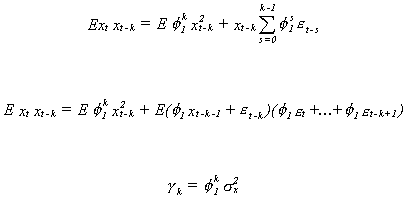Note that the autocovariances die out as k grows. The autocorrelation function is the autocovariance divided by the variance of the white noise term. Or,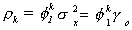.
Using the earlier Yule-Walker formulae for the partial autocorrelations we have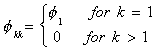For an AR(1) the autocorrelations die out exponentially and the partial autocorrelations exhibit a spike at one lag and are zero thereafter.

Example 2
Consider the AR(2)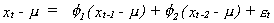The associated polynomial in the lag operator is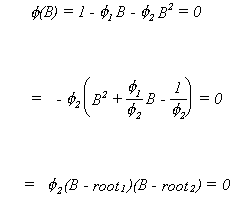The roots could be found using the quadratic formula. The roots are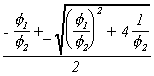When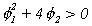the roots are real and as a consequence the series will decline exponentially in response to a shock.  When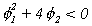the roots are complex and the series will appear as a damped sign wave.

The stationarity theorem imposes the following conditions on the AR coefficients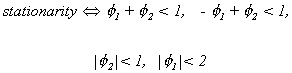The autocovariance for an AR(2) process, with zero mean, is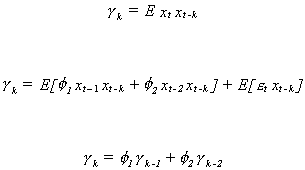Dividing through by the variance of xt gives the autocorrelation function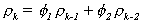Since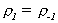we can write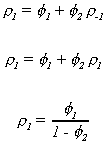Similarly for the second and third autocorrelations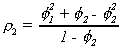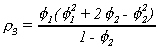The other autocorrelations are solved for recursively. Their pattern is governed by the roots of the second order linear difference equation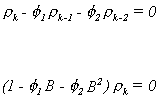If the roots are real then the autocorrelations will decline exponentially. When the roots are complex the autocorrelations will appear as a damped sine wave.
Using the Yule-Walker equations, the partial autocorrelations are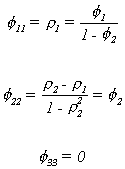Again, the autocorrelations die out slowly. The partial autocorrelation on the other hand is quite distinctive. It has spikes at one and two lags and is zero thereafter.

Theorem If xt is a stationary AR(p) process then it can be equivalently written as a linear filter model. That is, the polynomial in the backshift operator can be inverted and the AR(p) written as a moving average of infinite order instead.

Example
Suppose zt is an AR(1) process with zero mean;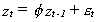. What is true for the current period must also be true for prior periods. Thus by recursive substitution we can write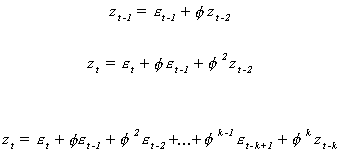Square both sides and take expectations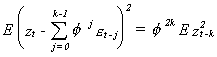the right hand side vanishes as k Ū Ĩ since ―f― < 1. Therefore the sum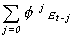converges to zt in quadratic mean. We can rewrite the AR(p) model as a linear filter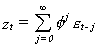that we know to be stationary.

The Autocorrelation Function
and Partial Autocorrelation Generally

Suppose that a stationary series zt with mean zero is known to be autoregressive. The autocorrelation function of an AR(p) is found by taking expectations of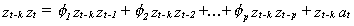and dividing through by the variance of zt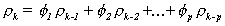This tells us that
rk is a linear combination of the previous autocorrelations. We can use this in applying Cramer's rule to (i) in solving for fkk. In particular we can see that this linear dependence will cause fkk = 0 for k > p. This distinctive feature of autoregressive series will be very useful when it comes to identification of an unknown series.

If you have either MathCAD or MathCAD Explorer then you can experiment interactivley with some fo the AR(p) ideas presented here.

Moving Average Models
Consider a dynamic model in which the series of interest depends only on some part of the history of the white noise term. Diagrammatically this might be represented asDefinition Suppose at is an uncorrelated sequence of i.i.d. random variables with zero mean and finite variance. Then a moving average process of order q, MA(q), is given by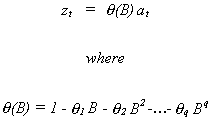Theorem: A moving average process is always stationary.
Proof: Rather than start with a general proof we will do it for a specific case. Suppose that zt is MA(1). Then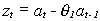. Of course, at has zero mean and finite variance. The mean of zt is always zero. The autocovariances will be given by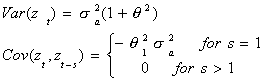You can see that the mean of the random variable does not depend on time in any way. You can also see that the autocovariance depends only on the offset s, not on where in the series we start. We can prove the same result more generally by starting with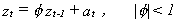, which has the alternate moving average representation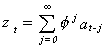. Consider first the variance of zt.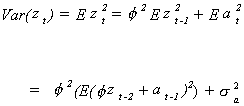By recursive substitution you can show that this is equal to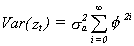The sum we know to be a convergent series so the variance is finite and is independent of time. The covariances are, for example,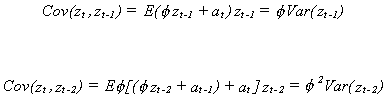You can also see that the auto covariances depend only on the relative points in time, not the chronological point in time. Our conclusion from all this is that an MA(Ĩ) process is stationary.
For the general MA(q) process the autocorrelation function is given by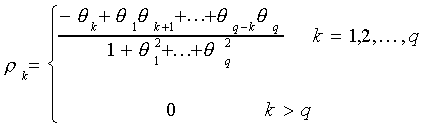The partial autocorrelation function will die out smoothly. You can see this by inverting the process to get an AR( ) process.

If you have either MathCAD or MathCAD Explorer then you can experiment interactively with some of the MA(q) ideas presented here.

Mixed Autoregressive - Moving Average Models

Definition Suppose at is an uncorrelated sequence of i.i.d. random variables with zero mean and finite variance. Then an autoregressive, moving average process of order (p,q), ARMA(p,q), is given by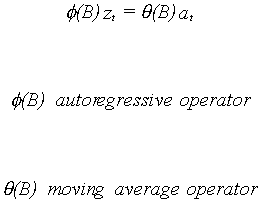The roots of the autoregressive operator must all lie outside the unit circle. The number of unknowns is p+q+2. The p and q are obvious. The 2 includes the level of the process, m, and the variance of the white noise term, sa2.

Suppose that we combine our AR and MA representations so that the model is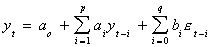(1)

and the coefficients are normalized so that bo = 1. Then this representation is called an ARMA(p,q) if the roots of (1) all lie outside the unit circle.
Suppose that the yt are measured as deviations from the mean so we can drop ao, then the autocovariance function is derived from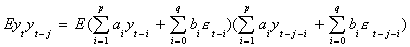if j>q then the MA terms drop out in expectation to give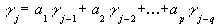That is, the autocovariance function looks like a typical AR for lags after q; they die out smoothly after q, but we cannot say how 1,2,…,q will look.
We can also examine the PACF for this class of model. The model can be written as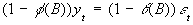We can write this as a MA(inf) process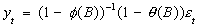which suggests that the PACF's die out slowly. With some arithmetic we could show that this happens only after the first p spikes contributed by the AR part.

Empirical Law In actuality, a stationary time series may well be represented by p Ģ 2 and q Ģ 2. If your business is to provide a good approximation to reality and goodness of fit is your criterion then a prodigal model is preferred. If your interest is predictive efficiency then the parsimonious model is preferred.

Experiment with the ARMA ideas presented above with a MathCAD worksheet.

Autoregressive Integrate Moving Average Models

MA filter AR filter Integrate filterSometimes the process, or series, we are trying to model is not stationary in levels. But it might be stationary in, say, first differences. That is, in its original form the autocovariances for the series might not be independent of the chronological point in time. However, if we construct a new series which is the first differences of the original series, this new series satisfies the definition of stationarity. This is often the case with economic data which is highly trended.

Definition Suppose that zt is not stationary, but zt - zt-1 satisfies the definition of stationarity. Also, at, the white noise term has finite mean and variance. We can write the model as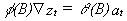This is named an ARIMA(p,d,q) model. p identifies the order of the AR operator, d identifies the power on Ņ, q identifies the order of the MA operator.
If the roots of f(B)Ņ lie outside the unit circle then we can rewrite the ARIMA(p,d,q) as a linear filter. I.e., it can be written as an MA(Ĩ). We reserve the discussion of the detection of unit roots for another part of the lecture notes.

Transfer Function Models

Consider a dynamic system with xt as an input series and yt as an output series. Diagrammatically we haveThese models are a discrete analogy of linear differential equations. We suppose the following relation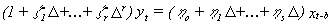where b indicates a pure delay. Recall that Ņ = (1-B). Making this substitution the model can be written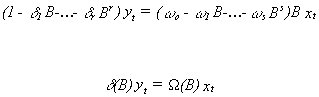If the coefficient polynomial on yt can be inverted then the model can be written as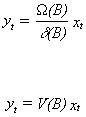V(B) is known as the impulse response function. We will come across this terminology again in our later discussion of vector autoregressive , cointegration and error correction models.

MODEL IDENTIFICATION
Having decided on a class of models, one must now identify the order of the processes generating the data. That is, one must make best guesses as to the order of the AR and MA processes driving the stationary series. A stationary series is completely characterized by its mean and autocovariances. For analytical reasons we usually work with the autocorrelations and partial autocorrelations. These two basic tools have unique patterns for stationary AR and MA processes. One could compute sample estimates of the autocorrelation and partial autocorrelation functions and compare them to tabulated results for standard models.

Definitions
Sample Mean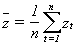Sample Autocovariance Function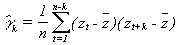Sample Autocorrelation Function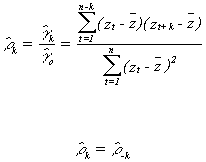The sample partial autocorrelations will be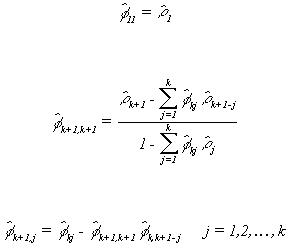Using the autocorrelations and partial autocorrelations is quite simple in principle. Suppose that we have a series zt, with zero mean, which is AR(1). If we were to run the regression of zt+2 on zt+1 and zt we would expect to find that the coefficient on zt was not different from zero since this partial autocorrelation ought to be zero. On the other hand, the autocorrelations for this series ought to be decreasing exponentially for increasing lags (see the AR(1) example above).
Suppose that the series is really a moving average. The autocorrelation should be zero everywhere but at the first lag. The partial autocorrelation ought to die out exponentially.
Even from our very cursory romp through the basics of time series analysis it is apparent that there is a duality between AR and MA processes. This duality may be summarized in the following table.

 AR(p) MA(q) ARMA(p,q) The stationary AR(p) f(B)zt = at can be represented as an MA of infinite order. The invertible MA(q) zt = q (B)at can be represented as an infinite order AR. Can be represented as either an AR() or MA(), conditional on roots. Autocorrelation rk Ū 0 but r đ 0 from f(B)=0. That is, the spikes in the correlogram decrease exp onentially. rk = 0 for k ģ q+1. There are spikes until lag q. Autocorrelations die out smoothly after q lags. Partial autocorrelation fkk = 0 for k ģ p+1. There are spikes in the correlogram at lags 1 through p. fkk Ū 0 but fkk đ 0 from q(B)=0. The spikes die out exponentially PACF's die out smoothly after p lags Stationarity All roots of f(B)=0 lie outside the unit circle. No restriction. Invertibility No restriction. All roots of q (B)=0 lie outside the unit circle.

Diagrams of the theoretical correlograms for AR and MA processes can be found in Hoff(1983, Pps. 59-71) or Wei(1990, Pp. 32-66). The correlograms for a stationary ARMA are somewhat more problematic. The autocorrelations show an irregular pattern of spikes through lag q, then the remaining pattern is the same as that for an AR process. The partial autocorrelations are irregular through lag p, then the PACs decay exponentially as in an MA process.